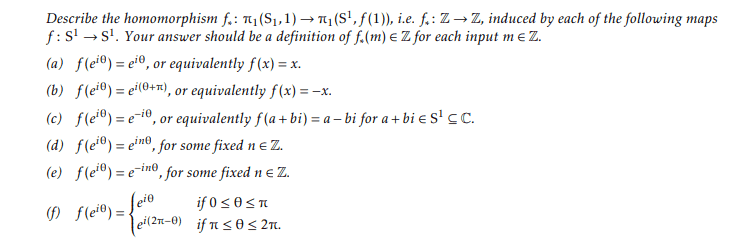# Describing homeomorphisms with the π1 function

• I
• Mikaelochi

#### Mikaelochi

TL;DR Summary
A problem from a topology class I took but never really understoodHere is what the problem looks like. The thing is I don't remember what π1is exactly and I don't really know much group theory or know what equivalence classes are. I remember learning some group theory fact that f*(n) = n*f*(1). So, I think (a) was just equal to m since f(1) = 1 and (b) was equal to -m. Anyway, I'd like to know if my answers to (a) and (b) are correct. And if I could get an explanation on how to even do (c) through (f). Thank you very much.

##S^1## is the unit circle.

##\pi_1(S^1, p)## is the fundamental group of the unit circle relative to point ##p##, which is the set of equivalence classes of closed loops in ##S^1## that start and finish at point ##p## on the circle.

A loop in ##S^1## is a continuous function ##g## from the unit interval ##[0,1]## to ##S^1##, for which ##g(0)=g(1)##, ie it finishes at the point where it began. It is a path together with specifying a direction and speed in which to traverse it.

An equivalence class of loops is the set of all loops that can be transformed into one another by smooth deformation - no cutting and reattaching allowed. The consequence of that is that the fundamental group of a set roughly relates to the number of 'holes' in the set, as a curve that goes right around a hole can't be smoothly deformed to not go around it.

So the fundamental group of the one-hole circle ##S^1## corresponds to the integers. To each integer ##m## corresponds a member of the fundamental group, an equivalence class, consisting of loops that go anti-clockwise around the circle a net ##m## times. The word 'net' indicates that if the loop doubles back on itself, the retraced path cancels out - eg if it goes anti-clockwise 5 times then clockwise twice, that is a net 3 and it corresponds to the integer 3.

Negative integers correspond to a net clockwise circling of the hole.

Use a simple loop to test each question - the simplest loop being a single anti-clockwise journey around the circle, given by ##g(t) = e^{2\pi i t}## for ##t\in]0,1]##. This belongs to the equivalence class corresponding to the integer 1. For each case you need to work out which integer the function ##h = f\circ g## (ie ##h(t) = f(g(t))##) corresponds to, ie how many times does the loop ##h## go around the circle and in which direction?

Once you've worked out what integer ##f## maps 1 to, you can work out ##f_*(m) = m\times f_*(1)##, using the homomorphism property. So if ##f_*(1)=-3## then ##f_*(-10) = 30##.

Some functions $f$ will reverse the direction (in which case 1 maps to a negative integer), and others will alter the number of rotations (in which case 1 maps to an integer with magnitude different from 1- either increased, or going to zero).

Your guess is correct for (a) but wrong for (b). The answer for (b) is the same as for (a). They both map 1 to 1, and hence map ##m## to ##m##. The only difference is the starting point, which is irrelevant. All that matters is number and direction of rotations.

You may find that the answer to (c) is negative, and the answer to (d) is not ##1## or ##-1##.

Last edited:
•Mikaelochi